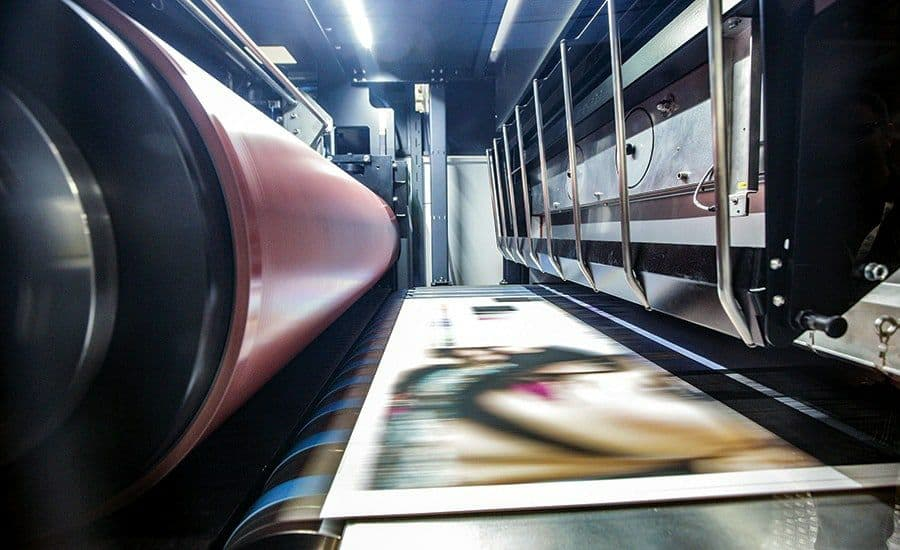CallbackNews
```
Conditional printed sheet
Conditional printed sheet (abbreviated conditional printed sheets) is a unit of measurement of the volume of a published book or article, equal to one side of a printed sheet of 60 × 90 cm format.To determine the volume of any printed publication in conditional printed sheets, one should calculate the ratio of physical printed sheets to sheets conditional.
The calculation formula is simple: divide the number of pages of the publication by the fraction of the sheet used in this publication format and multiply by the conversion factor.
The conversion factor of physical printed sheets to conventional is a number that shows how the area of ​​a physical printed sheet is related to the area of ​​a conventional printed sheet, or how many conventional printed sheets fit in physical printed sheets.
If the publication format is 70×100 / 16, then the conversion factor is calculated as follows:
Area of ​​the printed sheet of the edition: 70 cm x 100 cm = 7,000 cm2
The area of ​​a conventional printed sheet: 60 cm x 90 cm = 5 400 cm2
Conversion factor: 7,000 cm2 / 5,400 cm2 = 1.3
If the edition has 160 pages, then conditional printed sheets are calculated using the formula:
160 pages / 16 (proportion of the format 70×100 / 16) * 1.3 (conversion factor) = 13 conv. print l.
Conditional (standard) printed sheets are widely used to indicate the volumes of scientific and literary publications, to compare the printed volumes of different-format publications.
The first experimental printing house Kharkov – we print editions of standard and non-standard sizes! Write, call! Always in touch!
+38 (067) 543 56 55
+38 (099) 543 56 55
+38 (073) 545 56 55
www.exp-print.com.ua

```•## Conditional printed sheet

Conditional printed sheet (abbreviated conditional printed sheets) is a unit of measurement of the volume of a published book or article, equal to one side of a printed sheet of 60 × 90 cm format.To determine the volume of any printed publication in conditional printed sheets, one should calculate the ratio of physical printed sheets to sheets conditional.

The calculation formula is simple: divide the number of pages of the publication by the fraction of the sheet used in this publication format and multiply by the conversion factor.

The conversion factor of physical printed sheets to conventional is a number that shows how the area of ​​a physical printed sheet is related to the area of ​​a conventional printed sheet, or how many conventional printed sheets fit in physical printed sheets.

If the publication format is 70×100 / 16, then the conversion factor is calculated as follows:
Area of ​​the printed sheet of the edition: 70 cm x 100 cm = 7,000 cm2
The area of ​​a conventional printed sheet: 60 cm x 90 cm = 5 400 cm2
Conversion factor: 7,000 cm2 / 5,400 cm2 = 1.3

If the edition has 160 pages, then conditional printed sheets are calculated using the formula:
160 pages / 16 (proportion of the format 70×100 / 16) * 1.3 (conversion factor) = 13 conv. print l.
Conditional (standard) printed sheets are widely used to indicate the volumes of scientific and literary publications, to compare the printed volumes of different-format publications.

The first experimental printing house Kharkov – we print editions of standard and non-standard sizes! Write, call! Always in touch!
+38 (067) 543 56 55
+38 (099) 543 56 55
+38 (073) 545 56 55
www.exp-print.com.ua

© 2009-2022 The first experimental typography